# The STATESPACE Procedure

### Canonical Correlation Analysis

Subsections:

Given the order p, let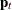be the vector of current and past values relevant to prediction of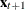: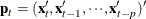Let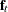be the vector of current and future values: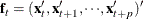In the canonical correlation analysis, consider submatrices of the sample covariance matrix ofand. This covariance matrix,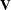, has a block Hankel form: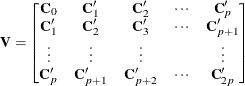#### State Vector Selection Process

The canonical correlation analysis forms a sequence of potential state vectors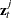. Examine a sequence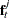of subvectors of, form the submatrix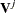that consists of the rows and columns ofthat correspond to the components of, and compute its canonical correlations.

The smallest canonical correlation ofis then used in the selection of the components of the state vector. The selection process is described in the following discussion. For more details about this process, see Akaike (1976).

In the following discussion, the notation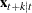denotes the wide sense conditional expectation (best linear predictor) of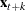, given all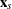with s less than or equal to t. In the notation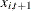, the first subscript denotes the ith component of.

The initial state vector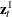is set to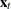. The sequenceis initialized by setting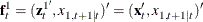That is, start by considering whether to add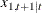to the initial state vector.

The procedure forms the submatrix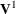that corresponds to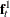and computes its canonical correlations. Denote the smallest canonical correlation ofas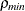. Ifis significantly greater than 0,is added to the state vector.

If the smallest canonical correlation ofis not significantly greater than 0, then a linear combination ofis uncorrelated with the past,. Assuming that the determinant of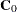is not 0, (that is, no input series is a constant), you can take the coefficient ofin this linear combination to be 1. Denote the coefficients ofin this linear combination as. This gives the relationship: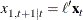Therefore, the current state vector already contains all the past information useful for predicting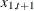and any greater leads of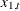. The variableis not added to the state vector, nor are any terms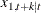considered as possible components of the state vector. The variable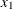is no longer active for state vector selection.

The process described foris repeated for the remaining elements of. The next candidate for inclusion in the state vector is the next component ofthat corresponds to an active variable. Components ofthat correspond to inactive variables that produced a zeroin a previous step are skipped.

Denote the next candidate as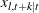. The vectoris formed from the current state vector andas follows: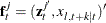The matrix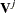is formed fromand its canonical correlations are computed. The smallest canonical correlation ofis judged to be either greater than or equal to 0. If it is judged to be greater than 0,is added to the state vector. If it is judged to be 0, then a linear combination ofis uncorrelated with the, and the variable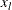is now inactive.

The state vector selection process continues until no active variables remain.

#### Testing Significance of Canonical Correlations

For each step in the canonical correlation sequence, the significance of the smallest canonical correlationis judged by an information criterion from Akaike (1976). This information criterion is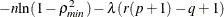where q is the dimension ofat the current step, r is the order of the state vector, p is the order of the vector autoregressive process, andis the value of the SIGCORR= option. The default is SIGCORR=2. If this information criterion is less than or equal to 0,is taken to be 0; otherwise, it is taken to be significantly greater than 0. (Do not confuse this information criterion with the AIC.)

Variables in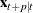are not added in the model, even with positive information criterion, because of the singularity of. You can force the consideration of more candidate state variables by increasing the size of thematrix by specifying a PASTMIN= option value larger than p.

#### Printing the Canonical Correlations

To print the details of the canonical correlation analysis process, specify the CANCORR option in the PROC STATESPACE statement. The CANCORR option prints the candidate state vectors, the canonical correlations, and the information criteria for testing the significance of the smallest canonical correlation.

Bartlett’sand its degrees of freedom are also printed when the CANCORR option is specified. The formula used for Bartlett’sis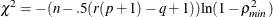with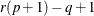degrees of freedom.

Figure 28.12 shows the output of the CANCORR option for the introductory example shown in the Getting Started: STATESPACE Procedure.

proc statespace data=in out=out lead=10 cancorr;
var x(1) y(1);
id t;
run;


Figure 28.12: Canonical Correlations Analysis

The STATESPACE Procedure
Canonical Correlations Analysis

x(T;T) y(T;T) x(T+1;T) Information Criterion Chi-Square DF
1 1 0.237045 3.566167 11.4505 4

New variables are added to the state vector if the information criteria are positive. In this example,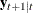and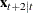are not added to the state space vector because the information criteria for these models are negative.

If the information criterion is nearly 0, then you might want to investigate models that arise if the opposite decision is made regarding. This investigation can be accomplished by using a FORM statement to specify part or all of the state vector.

#### Preliminary Estimates of F

When a candidate variableyields a zeroand is not added to the state vector, a linear combination ofis uncorrelated with the. Because of the method used to construct thesequence, the coefficient ofincan be taken as 1. Denote the coefficients ofin this linear combination as.

This gives the relationship: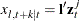The vectoris used as a preliminary estimate of the first r columns of the row of the transition matrix
corresponding to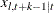.